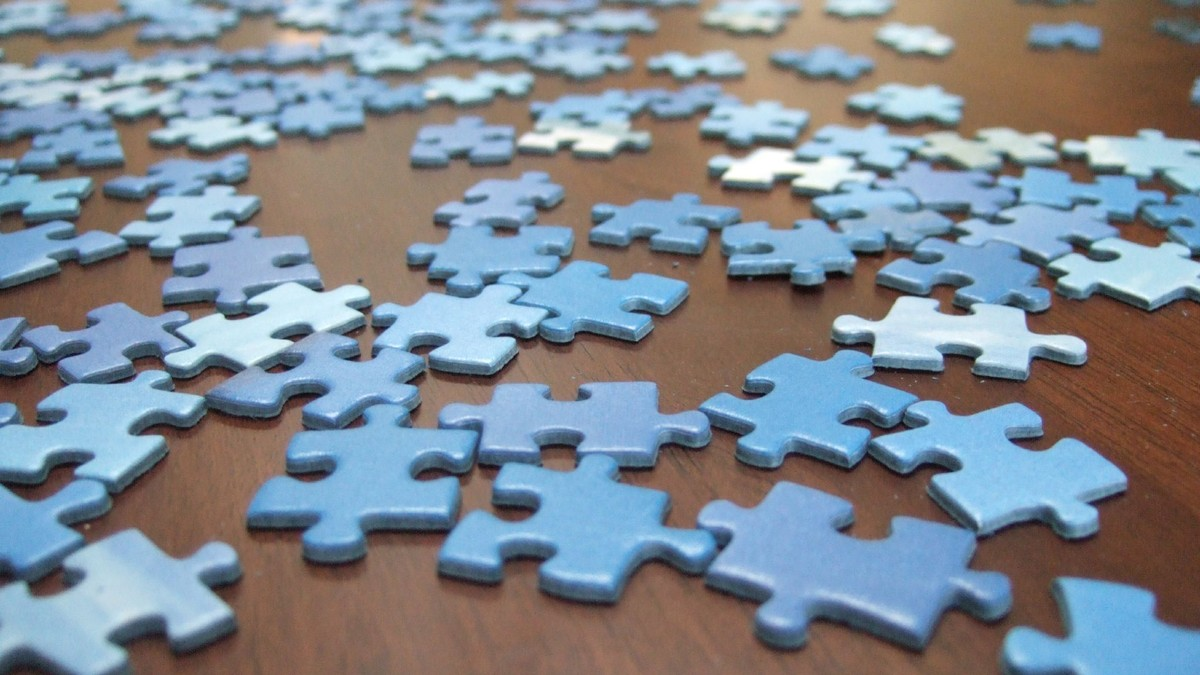Connect with us

# Why Jigsaw Puzzles Almost Never Have the Number of Pieces it Says on the BoxPublished

onThis piece was originally published on God Plays Dice.

Patrick Honner tweeted a few days ago:

So let’s say that a number is a “puzzle number” if it’s of the form m x n with m ≤ n ≤ 2m — that is, a puzzle has to have an aspect ratio between 1 (square) and 2. (The choice of 2 is arbitrary here, but any other constant would be more arbitrary.) We can easily work out the first few puzzle numbers:

2 = 1×2, 4 = 2×2, 6 = 2×3, 8 = 2×4, 9 = 3×3

which is enough to find them in the OEIS: that’s A071562, defined as “Numbers n such that the sum of the middle divisors of n (A071090) is not zero.” (What’s a “middle divisor”, you ask? It’s a divisor of n that’s between (square root n/2) and (square root 2n.)) Titling it…